• 五参数拟合曲线
2021-05-20 14:25:01

《4参数拟合汇总10页》由会员分享，可在线阅读，更多相关《4参数拟合汇总10页(10页珍藏版)》请在人人文库网上搜索。

1、曲线拟合、回归模型介绍一、直线拟合回归：直线回归是最简单的回归模型,也是最基本的回归分析方法， 将所有的测试点拟合为一条直线，其方程式为：y=a+bx 二、二次多项式拟合回归：二次多项式成抛物线状，开口向下或者向上，在很多ELISA实验中，拟合近似于二次多项式的升段或者降段，由于曲线的特性，同一个浓度值在曲线图上可能表现出没有对应的OD值、有一个OD值，或者两个OD值，所以使用二次多项式拟合时，最好保证取值的范围都落在曲线的升段或者降段，否则哪怕是相关系数很好也很可能与实际的值不一致。其方程式为：y = a + b x + c x2 ，形状如下图：三、三次多项式拟合回归：三次多项式像倒状的S形。

2、，在实验结果刚好在曲线的升段或者降段的时候，效果还可以，但是对于区间较广的情形, 由于其弯曲的波动，三次方程拟合模拟不一定很好.跟二次方程拟合一样，看曲线的相关系数的同时也要看计算的点在曲线上的分布，这样才算出理想的结果，本软件计算值时，选择性的取相对于浓度或者OD值，比较符合实际的那个结果，而没有将多个结果列出。方程式为：y = y = a + b x + c x2 + d x3 ，形状如下图：四、半对数拟合回归：半对数拟合即将浓度值取对数值，然后再和对应的OD值进行直线回归，理想的状态下，在半对数坐标中是一条直线，常用于浓度随着OD值的增加或者减低呈对数增加或者减少的情况，即浓度的变化比O。

3、D值的变化更为剧烈。在ELISA实验中较常用(有很多用EXCEL画图时，也常使用半对数)方程式为：y = a lg(x) + b ，形状如下图(注意其X轴是对数坐标)：五、Log-Log拟合回归：Log-Log拟合和半对数相似,只是将OD值和对应的浓度值均取对数，然后再进行直线回归，方程式为：lg(y) = a lg(x) + b ，形状如下图：六、Logit-log 直线回归：Logit-log 则是免疫学检测中的模型, 可用于竞争法. 它最早用于 RIA, 但在 ELISA 中也是可以应用的. Logit 变换源于数学中的 Logistic 曲线.在竞争 RIA 及 ELISA 中, 当竞。

4、争性反应物为 0 时结合率为 100%, 如果某一浓度下结合率为 B,B=OD/OD(0),在对B进行 Logit 变换:y=lnB/(1-B) ,之后y与浓度的对数成线性关系，即:y = a + b lg x方程式为：lg(y) = a lg(x) + b 就得到了Logit-log 直线回归模型，这个模型一般适用于竞争法的拟合，所以拟合时要求只有少有一个零浓度测试的OD值，并且此值为整个反应的最大值(也就是我们常说的至少要做一个空白对照)。七、四参数拟合回归：四参数方程的表达式为：它不仅限于竞争法, 实际上夹心法也可以用它。它的形状, 根据情况, 可能是一个单调上升的类似指数, 对数, 或。

5、双曲线的曲线, 也可能是一个单调下降的上述曲线, 还可以是一条 S 形曲线。 它要求 X 值不能小于0 (因为指数是实数, 故有此要求)。 在很多情况下它都可以拟合 ELISA 的反应曲线, 所以它也成了 ELISA 中应用最广的模型之一。八、三次样条插值：早期工程师制图时，把富有弹性的细长木条(所谓样条)用压铁固定在样点上，在其他地方让它自由弯曲，然后沿木条画下曲线。成为样条曲线，三次样条插值(简称Spline插值)是通过一系列形值点的一条光滑曲线，数学上通过求解三弯矩方程组得出曲线函数组的过程。所以三次样条插值实际上各个测试点间的每一段都是一个三次方程，并对两端都进行平滑处理，得到的一组三次方程组。本软件的算法中的边界条件取的是自然边界(即边界点的导数为0,)这样处理出来的曲线更符合ELISA的实验结果，在数据点较多时，其拟合的效果也和实际结果非常吻合。现在有些自动化的分析仪器中，比如某些型号的全自动化学发光分析仪，计算结果就是使用三次样条插值进行结果的处理的。九、点对点计算：顾名思义，点对点就是将测试点画在坐标上，然后依次用直线连起来，然后依照浓度或者OD值，求出其在某一段直线上的OD值或者浓度值，是一种较为粗糙的拟合方法，在数据较为密集时结果还算可以。4参数拟合 c语言
更多相关内容
• 特别是，五参数对数回归或5PL非线性回归模型通常用于生物测定或免疫测定（例如ELISA，RIA，IRMA或剂量React曲线）中的曲线拟合分析。 标准剂量React曲线有时称为五参数逻辑方程。 它的特点是其经典的“S”或 S 形...matlab
• ## 【Matlab】曲线拟合

千次阅读 2022-06-12 11:27:16
调用格式：[P,S,mu]=polyfit(X,Y,m)[P,S]=polyfit(X,Y,m)P=polyfit(X,Y,m)参数解释：根据样本数据X和Y，产生一个m次多项式系数向量P及其在采样点误差数据S，mu是一个二元向量，mu(1)是mean(X)，而mu(2)是std(X)。...

# 曲线拟合

插值与拟合的区别：

1. 实现方法：插值要求曲线穿过样本点，而拟合不需要穿过样本点，只要求总体误差最小。
2. 结果形式：插值是分段逼近样本点，没有同一的逼近函数；函数拟合则用一个函数去逼近，有完整的表达式。
3. 侧重点：插值可以用于估计区间内某些点对应的函数值；拟合不仅可以估计区间内的点，也可以预测区间外的点。
4. 应用场合：插值多用于精确数据集；拟合多用于统计数据集。

polyfit函数用于一元多次曲线拟合（亦多项式拟合），形如： y = a x 5 + b x 4 + c x 3 + d x 2 + e x + f y=ax^5+bx^4+cx^3+dx^2+ex+f ，我们已知 x x y y 样本数据，利用此函数求解参数（系数） a a b b c c d d e e f f

regress函数用于一元或多元线性回归（拟合），形如： y = a x 1 + b x 2 + c x 3 + d x 4 + e y=ax_1+bx_2+cx_3+dx_4+e ，我们已知 x 1 x_1 x 2 x_2 x 3 x_3 x 4 x_4 y y 样本数据，利用此函数求解参数（系数） a a b b c c d d e e

## polyfit函数

调用格式：

• [P,S,mu]=polyfit(X,Y,m)

• [P,S]=polyfit(X,Y,m)

• P=polyfit(X,Y,m)

参数解释：

根据样本数据X和Y，产生一个m次多项式系数向量P及其在采样点误差数据S，mu是一个二元向量，mu(1)是mean(X)，而mu(2)是std(X)。

x=0: 0.1: 1;
y=[-0.447, 1.978, 3.11, 5.25, 5.02, 4.66, 4.01, 4.58, 3.45, 5, 35];
p = polyfit(x, y, 3) % 三次多项式拟合
xx = 0: 0.01 : 1;
yy = polyval(p, xx) ; % 根据系数向量p计算在xx点处的函数值
plot(xx, yy, '-b', x, y, 'markersize', 20)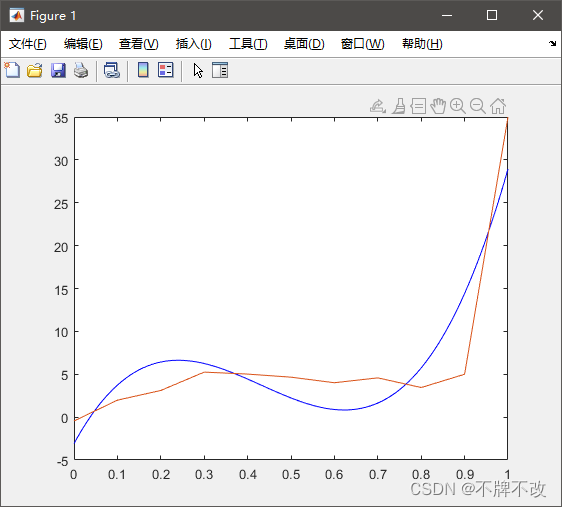当还需要进行线性回归分析时，可以再利用corrcoef函数获取相关系数。

## regress函数

在matlab的regress函数中置信区间bint、rint以及stats后面三个值全都为无穷大，这说明数据不服从线性关系，应考虑用非线性拟合函数来拟合。

调用格式：

• [B,BINT,R,RINT,STATS] = regress(Y,X)
• [B,BINT,R,RINT] = regress(Y,X)
• [B,BINT,R] = regress(Y,X)
• [B,BINT] = regress(Y,X)
• B = regress(Y,X)

参数解释：

调用该函数要保证目标函数的形式已知，比如目标函数为： y = a x 1 + b x 2 + c x 3 + d x 4 + e y=ax_1+bx_2+cx_3+dx_4+e ，或者是 y = a x 1 2 + b x 2 2 + c x 1 + d x 2 + e x 1 × x 2 + f y = ax_1^2+bx_2^2+cx_1+dx_2+ex_1×x_2+f 这样的多元多次多项式。传入参数，调用该函数后得到拟合出的系数值。

• x是一个矩阵。矩阵的每一个样本，即行数等于样本数，每个样本的每一列表示该样本在多项式中每一项除去系数之外的值。
• y是一个列向量。向量维度与矩阵行数一致，同为样本数，每一个值表示每个样本对应的函数值。

该函数根据多个样本的 x i x_i y y 拟合出多项式的系数。

返回值解释：

• B：回归系数，即未知参数，B(1)为常数项，B(2~……)依次为X每一列（从第二列起）对应的项的系数；
• BINT：回归系数的置信区间。（置信区间：当给出某个估计值的95%置信区间为[a, b]时，可以理解为我们有95%的信心可以说样本的平均值介于a到b之间，而发生错误的概率为5%）
• R：残差（残差是指观测值与预测值（拟合值）之间的差，即是实际观察值与回归估计值的差）
• RINT：残差的置信区间。
• STATS：用于检验回归模型的统计量。有4个数值：判定系数 R 2 R^2 （度量拟合优度的统计量，R²的值越接近1，说明回归直线对观测值的拟合程度越好）， F F 统计量观测值，检验的 p p 的值（ p < 0.05 p<0.05 时回归模型成立），误差方差的估计。
x1=[3.91 6.67 5.33 5.56 6.12 7.92 5.82 5.5 5.59 6.12 6.68 6.93]';
x2=[9.43 14.5 15.8 19.8 17.4 23.8 31.6 37.1 36.4 32.2 36.6 41.3]';
X=[ones(12,1), x1, x2];
Y=[280 338 405 432 452 582 596 602 606 621 629 656]';

[b,bint,r,rint,stats] = regress(Y,X)
rcoplot(r,rint) % 绘制残差图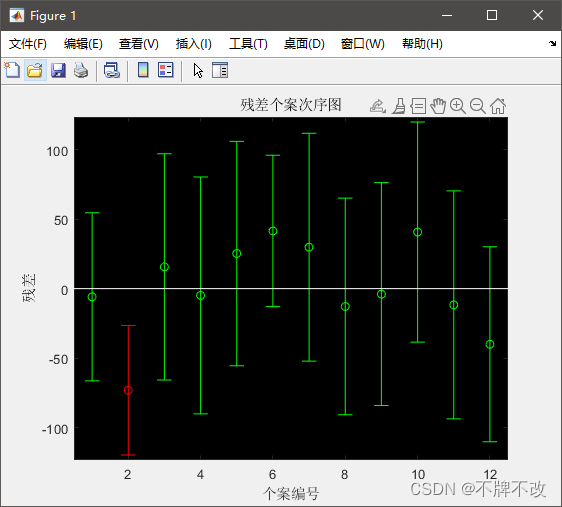残差图：残差图中圆圈是每个数据点的实际残差，横线区间是残差置信区间，置信区间穿过原点说明方程拟合的很好；未通过原点，可视为异常点，比如上图中显示第二组数据未通过原点，因此第二组数据拟合结果较差。

之后可将第二组数据去除后再次进行拟合，得到的结果更为准确。

%%  目标函数：y=Ax1^2+Bx2^2+Cx1+Dx2+Ex1*x2+F  （这是一个二次函数，两个变量，大写的字母是常数）
format long;

y=[7613.51 7850.91 8381.86 9142.81 10813.6 8631.43 8124.94 9429.79 10230.81 10163.61 9737.56 8561.06 7781.82 7110.97]';
x1=[7666 7704 8148 8571 8679 7704 6471 5870 5289 3815 3335 2927 2758 2591]';
x2=[16.22 16.85 17.93 17.28 17.23 17 19 18.22 16.3 13.37 11.62 10.36 9.83 9.25]';
X=[ones(size(y)) x1.^2 x2.^2 x1 x2 x1.*x2]; % 构造X矩阵！！！

[b,bint,r,rint,stats] = regress(y,X)


结果如下：

b =

1.0e+04 *

-1.353935450267780
0.000000089381408
-0.005811190715467
-0.000605427789545
0.479983626458515
-0.000037869040292

bint =

1.0e+04 *

-2.621944842897225  -0.085926057638335
0.000000034253753   0.000000144509063
-0.027588831662544   0.015966450231609
-0.001309493882546   0.000098638303455
0.119564693553897   0.840402559363132
-0.000105954336341   0.000030216255756

r =

1.0e+02 *

-4.397667358984126
-2.361417286008764
-1.434643909138249
-5.904203974279353
7.511701773844997
5.570806699070599
-2.447861341816779
0.494622057474844
6.376995507987613
-6.789520765534544
2.744335484633611
1.578124015815701
-0.803533566911865
-0.137737336155596

rint =

1.0e+03 *

-1.219619853471144   0.340086381674319
-1.426253867770768   0.953970410569015
-0.919089416302223   0.632160634474573
-1.568776909577359   0.387936114721488
0.111430783412043   1.390909571356956
-0.533006860832905   1.647168200647025
-1.168898277755904   0.679326009392548
-0.977546779130818   1.076471190625786
-0.398546999643731   1.673946101241254
-1.439044988776064   0.081140835669156
-0.919162439561023   1.468029536487746
-1.088788822632291   1.404413625795431
-1.271236485719865   1.110529772337492
-1.017369947314269   0.989822480083149

stats =

1.0e+05 *

0.000008444011951   0.000086828553270   0.000000043344434   3.162249735298930


参数X的第一列为全1列向量，从第二列开始分别为每一个系数对应项对应样本数据构成的列向量，本题的目标函数是个二元二次的，由于regress函数只能解决线性问题，因此我们将 x 1 2 x_1^2 看作第一项， x 2 2 x_2^2 看作第二项， x 1 x_1 看作第三项， x 2 x_2 看作第四项， x 1 x 2 x_1x_2 看作第五项，这样就相当于一个五元一次的目标函数了。

b为对应的参数 b(1)为F(最后那个常数项) ，b(2)为A（第一个参数），b(3)为B，b(4)为C，b(4)为D，b(5)为E。

bint为b的95%置信区间。

stats的第三个参数为F检测的p值，p值很小（p<0.05），说明拟合模型有效。

## 应用

已知随机性参数与多样性度量和与收敛性度量之间的关系如下表，多样性与收敛性同样重要，问选取随机参数的平衡点。

表1 随机性参数与多样性度量之间的关系

 x 0.03 0.06 0.09 0.12 0.15 0.18 0.21 0.24 0.27 0.3 y1 0.01 0.01 0.02 0.03 0.06 0.07 0.13 0.17 0.25 0.37

表2 随机性参数与收敛性度量之间的关系

 x 0.03 0.06 0.09 0.12 0.15 0.18 0.21 0.24 0.27 0.3 y1 0.85 0.76 0.68 0.62 0.54 0.52 0.5 0.49 0.48 0.47
x=0.03:0.03:0.3;
y1=[0.01,0.01,0.02,0.03,0.06,0.07,0.13,0.17,0.25,0.37];
y2=[0.85,0.76,0.68,0.62,0.56,0.52,0.49,0.46,0.43,0.39];
plot(x,y1,'*',x,y2,'o');
legend('多样性','收敛性')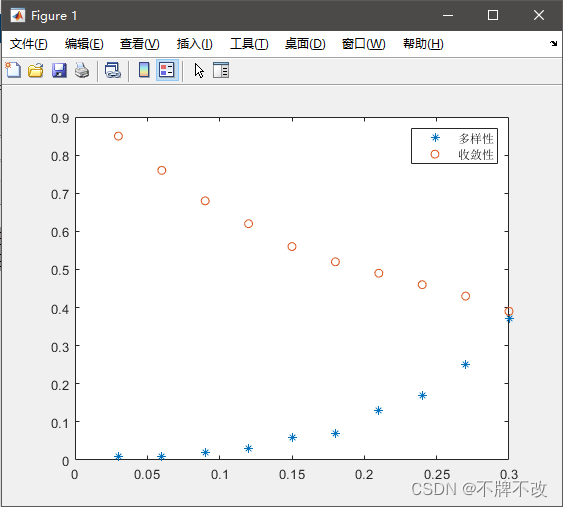问题分析：

随机性参数的增长导致多样性增加，收敛性降低；两者同等重要，则取平衡点；平衡点最佳位置是多样性和收敛性相等的地方。

解决方案：

第一步：分别对多样性和收敛性进行拟合，得到拟合曲线。

第二步：找到两曲线的交点。

p1=polyfit(x,y1,2);
p2=polyfit(x,y2,2);
p=p1-p2;
xi=roots(p); % -1.14148334023966	0.316172995412073
xj=0:0.03:0.36;
yj1=polyval(p1,xj);
yj2=polyval(p2,xj);
yi=polyval(p1,xi(2))
plot(x,y1,'*',x,y2,'o',xj,yj1,xj,yj2,xi(2),yi,'rp');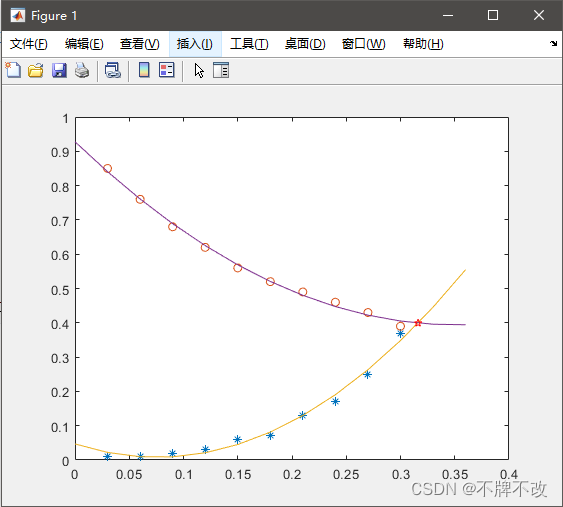## lsqlin函数

由于这个函数用的比较少，网上相关资料也就比较少，因此下面部分思路比较主观。

具有边界或线性约束的线性最小二乘求解器。

求解以下形式的最小二乘曲线拟合问题：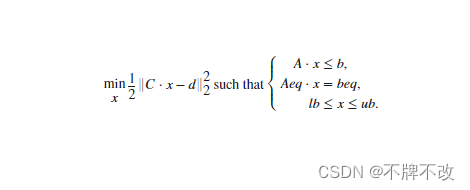详见，其实官方的也不详细，样例很差

这里我就举个最简单的例子：

%% y=ax^2+bxsinx+cx^3
xdata = [3.6,7.7,9.3,4.1,8.6,2.8,1.3,7.9,10.0,5.4];
ydata = [16,150.5,260.1,22.5,206.5,9.9,2.7,165.5,325.0,54.5];
C =[ xdata'.^2, xdata'.*sin(xdata'),xdata'.^3]; d = ydata';
[x, resnorm, residual] = lsqlin(C, d);

%% 绘图
xi = 0:0.1:11;
f = @(c, x) c(1).*x.^2 + c(2).*x.*sin(x) + c(3).*x.^3;
plot(xdata, ydata, '*', xi, f(x, xi))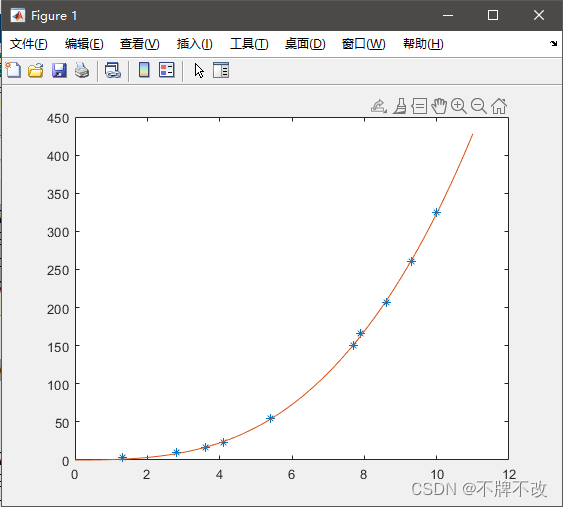函数的缺点：当上边界（ub）或下边界（lb）或Aeq或beq中的限制是对非多项式项进行的时，这些限制矩阵是很难构造出来的。比如，上面的代码中将 x 2 x^2 x s i n x xsinx x 3 x^3 分别看作第一、二、三项，因此约束条件矩阵也必须是对这三项的约束，但是若题目只给出了对 x x 的限制呢？若题目只给出了对 x e x l g x xe^xlgx 的限制呢？很难转化成对每一项的约束条件。

不知道如何处理”某些项有上下界的限制，而其他项没有上下界的限制“的情况。

对于这个函数所知甚少，且网上相关内容太少了。

## lsqcurvefit函数

最小二乘法求解非线性拟合问题！！！

调用格式：

• x = lsqcurvefit(fun,x0,xdata,ydata)

• x = lsqcurvefit(fun,x0,xdata,ydata,lb,ub)

• x = lsqcurvefit(fun,x0,xdata,ydata,lb,ub,options)

• [x,resnorm,residual,exitflag] = lsqcurvefit(…)

参数解释：

• x0为待求参数（初始解向量），初始变量的选取影响最终解
• xdata，ydata为用于拟合的数据
• lb、ub为解向量的下界和上界lb≤x≤ub，若没有指定界，则lb=[ ]，ub=[ ]
• fun为待拟合函数，计算x处拟合函数值，其参数一般有两类，一类是目标函数的参数，一类是目标函数的未知数

返回值解释：

• x为待求参数向量
• resnorm=sum ((fun(x,xdata)-ydata).^2)，即在x处残差的平方和
• residual=fun(x,xdata)-ydata，即在x处的残差
• exitflag为终止迭代的条件
%% y=tcos(kx)e^(wx)
x=[0,0.4,1.2,2,2.8,3.6,4.4,5.2,6,7.2,8,9.2,10.4,11.6,12.4,13.6,14.4,15]';
y=[1,0.85,0.29,-0.27,-0.53,-0.4,-0.12,0.17,0.28,0.15,-0.03,-0.15,-0.07,0.059,0.08,0.032,-0.015,-0.02]';
f= @(c,x) c(1)*cos(c(2)*x).*exp(c(3)*x);
c0= [0 0 0];
[c, fval]= lsqcurvefit(f, c0, x, y);
xx=0:0.1:20;
yy=f(c, xx);
plot(x, y, 'r*', xx, yy, 'b-');
disp(c);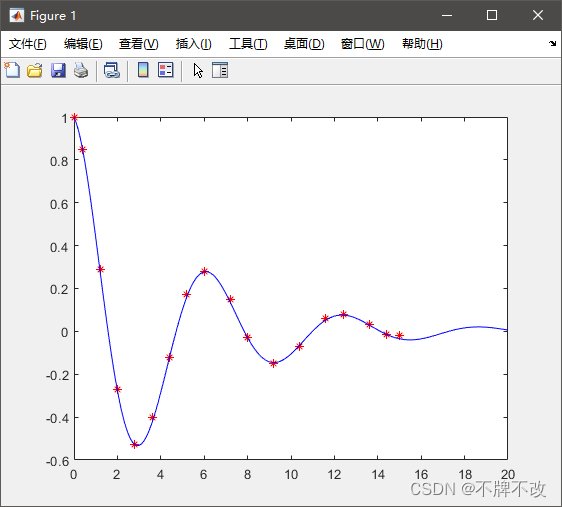注意：这里定义的函数比较特别，待拟合参数也要作为函数的参数传到函数中，我们将待拟合参数视为一个向量传入函数的目的是使函数的形式更加简洁。

xdata = [3.6,7.7,9.3,4.1,8.6,2.8,1.3,7.9,10.0,5.4];
ydata = [16,150.5,260.1,22.5,206.5,9.9,2.7,165.5,325.0,54.5];
c0=[ 0 0 0];
f_h=@(c, x) c(1)*x.^2 + c(2)*x.*sin(x) + c(3)*x.^3;
[c, resnorm, r]=lsqcurvefit(f_h, c0, xdata, ydata);

%% 绘图
xx=0:0.1:11;
yy=f_h(c, xx);
plot(xdata, ydata, 'r*', xx, yy, 'b-');
disp(c);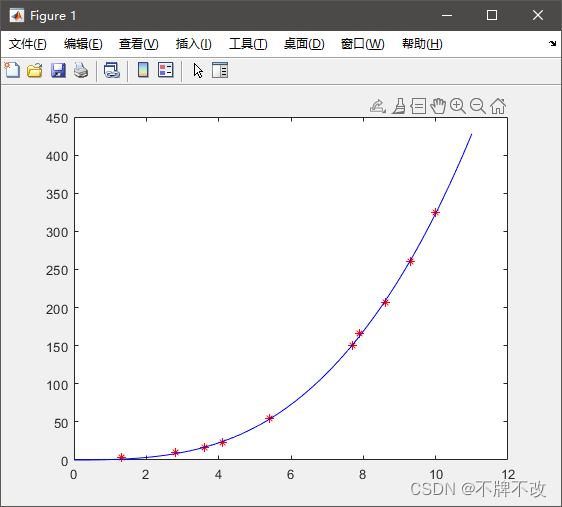## fittype函数和fit函数

自行学习，用的较少，需要时直接百度即可（对于打比赛的而言）。

展开全文matlab
• 这时一般要通过增加特征项或者减少正则化参数来改进模型。而过拟合一般是由于模型使用了太多的特征引起的，使得模型将部分数据的“特性”也学习到了，导致模型的泛化能力较弱。这时一般要通过删减特征项或...

在机器学习领域，模型的欠拟合问题和过拟合问题一直都是我们关注的重点，正确的诊断出你的模型属于哪一类问题对改善模型至关重要。

所谓欠拟合一般是指模型没有很好的抓住数据的特征，没有对数据进行很好的拟合，使得偏差较大。这时一般要通过增加特征项或者减少正则化参数来改进模型。

而过拟合一般是由于模型使用了太多的特征引起的，使得模型将部分数据的“特性”也学习到了，导致模型的泛化能力较弱。这时一般要通过删减特征项或者增大正则化参数来改进模型。

本文通过单变量的线性回归来说明应该通过哪些操作来诊断模型是属于欠拟合还是过拟合。

对于单变量的线性回归，我们最简单的一个模型就是一次方程。我们的假设函数如下。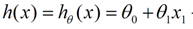利用这个模型来拟合数据，绘制的拟合效果图如下。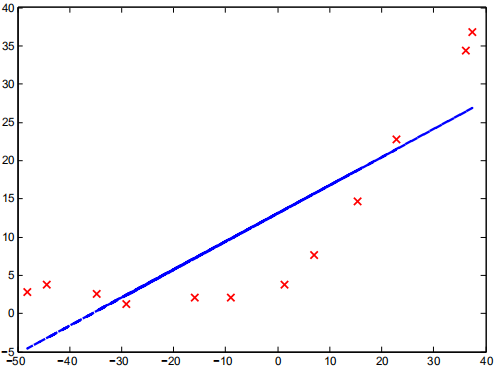不难看出，这个模型对数据的拟合效果不好，我们可以直观的判定这个模型存在欠拟合问题。但能不能通过什么有效的方法判断一个模型到底是存在欠拟合还是过拟合的问题呢。

答案是肯定的，我们可以绘制这个模型的学习曲线，通过学习曲线的形态来判断。所谓学习曲线就是训练集得分和验证集得分随着训练样本数的增大而变化的曲线。

当模型出现欠拟合和过拟合情况时，学习曲线一般有不同形状，如下图所示。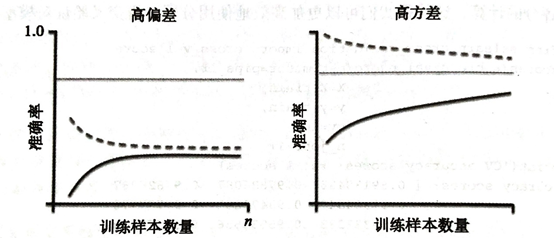欠拟合情况：随着训练样本数增大，训练集得分和验证集得分收敛，并且两者的收敛值很接近。

过拟合情况随着训练样本数增大，训练集得分和验证集得分相差还是很大。

下面利用sklearn包绘制单变量线性回归的Python代码如下。

import scipy.io as sio
from sklearn.model_selection import learning_curve
from sklearn import linear_model
import matplotlib.pyplot as plt
import numpy as np

# 读取以MATLAB矩阵存储的数据集

X_train = data['X']
X_cv = data['Xval']
X_train = np.concatenate((X_train,X_cv),axis=0)

Y_train = data['y']
Y_cv = data['yval']
Y_train = np.concatenate((Y_train,Y_cv),axis=0)
Y_train = Y_train.reshape([1, len(Y_train)])

reg = linear_model.Ridge(alpha=0)
train_sizes, train_scores, valid_scores = learning_curve(reg, X_train, Y_train, train_sizes=range(1,25,1), cv=4)
train_std=train_scores.mean(axis=1)
test_std=valid_scores.mean(axis=1)
plt.plot(train_sizes,train_std,color='red')
plt.plot(train_sizes,test_std,color='blue')
plt.xlabel('')
plt.show()

得到的学习曲线如下。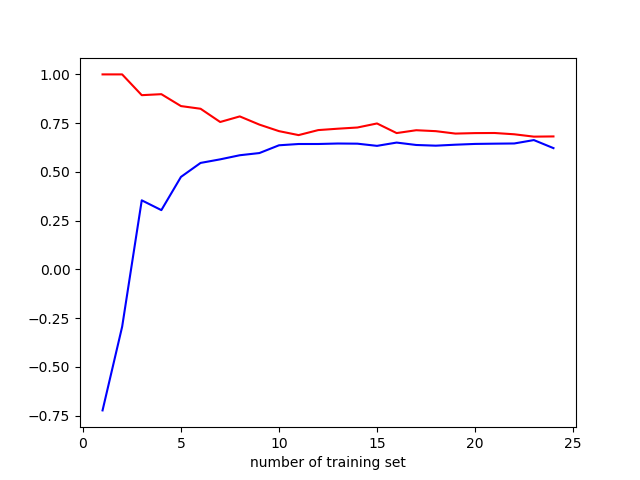通过观察学习曲线，可以判断模型存在欠拟合问题。我们试着利用多项式回归来改进模型。此时模型的假设函数形式如下。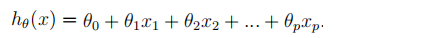其中Xp=X1的p次方。

我们可以用p=1、2、...、8依次试探，但有什么策略选出最好的p值吗？答案是肯定的，我先给出如下一张图。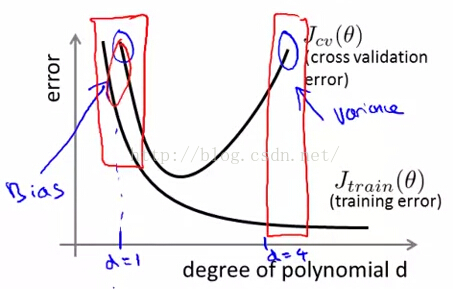这幅图描绘的是训练误差和验证误差随着多项式次数的增加而变化的图。当d很小的时候，训练误差和验证误差都很大，并且很接近，此时模型存在欠拟合问题；当d较大时，训练误差很小，但验证误差还是很大，此时模型存在过拟合问题。我们要取中间较均衡的d作为多项式的次数。

利用sklearn绘制的图如下所示。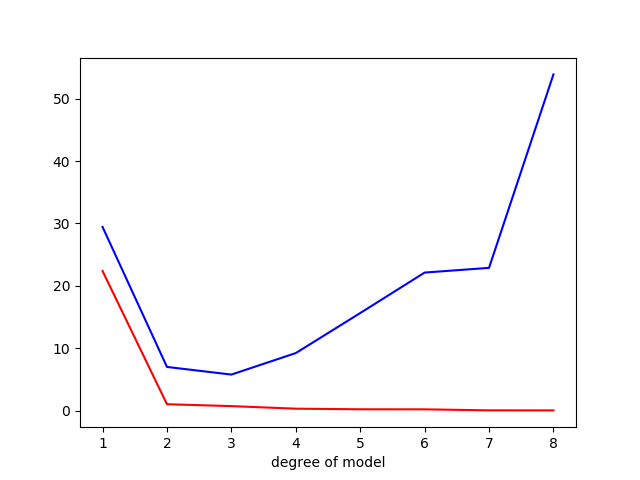通过上图的观察，我们可以取3作为多项式模型的次数。此时模型的拟合效果如下图所示。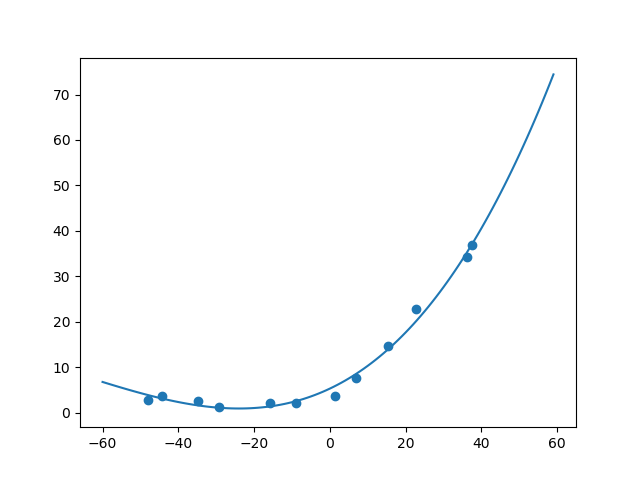好了，本次的文章就写到这里了。展开全文overfit underfit
•  全参考图像质量评价中，PSNR的输出取值范围是0～100 dB，想要把其输出范围映射到0～1区间的话，参考的方法，用下面的式子来拟合，总共有5个参数。 下面的代码中，xdata表示PSNR的数值，ydata表示对应的0～1...

example：xdata是输入，ydata是输出

import numpy as np
import matplotlib.pyplot as plt
from scipy.optimize import curve_fit

def func(x, a, b, c):
return a * np.exp(-b * x) + c

# Define the data to be fit with some noise:
xdata = np.linspace(0, 4, 50)
y = func(xdata, 2.5, 1.3, 0.5)
np.random.seed(1729)
y_noise = 0.2 * np.random.normal(size=xdata.size)
ydata = y + y_noise
plt.plot(xdata, ydata, 'b-', label='data')

# Fit for the parameters a, b, c of the function func:
popt, pcov = curve_fit(func, xdata, ydata)
print(popt)
plt.plot(xdata, func(xdata, *popt), 'r-',
label='fit: a=%5.3f, b=%5.3f, c=%5.3f' % tuple(popt))

# Constrain the optimization to the region of 0 <= a <= 3, 0 <= b <= 1 and 0 <= c <= 0.5:
popt, pcov = curve_fit(func, xdata, ydata, bounds=(0, [3., 1., 0.5]))
print(popt)
plt.plot(xdata, func(xdata, *popt), 'g--',
label='fit: a=%5.3f, b=%5.3f, c=%5.3f' % tuple(popt))

plt.xlabel('x')
plt.ylabel('y')
plt.legend()
plt.show()

全参考图像质量评价中，PSNR的输出取值范围是0～100 dB，想要把其输出范围映射到0～1区间的话，参考的方法，用下面的式子来拟合，总共有5个参数。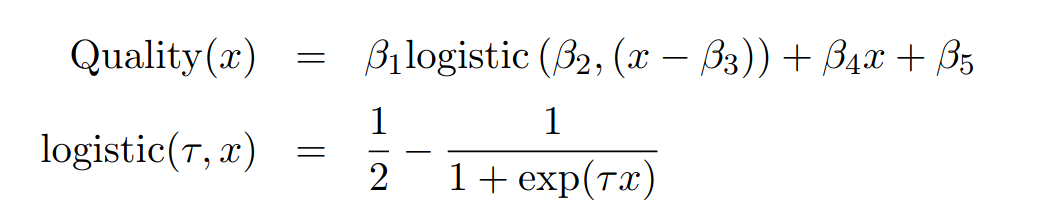下面的代码中，xdata表示PSNR的数值，ydata表示对应的0～1范围数值。

import numpy as np
import matplotlib.pyplot as plt
from scipy.optimize import curve_fit

def func(x, a, b, c, d, e):
logist = 0.5 - 1/(1+np.exp(b * (x-c)))
return a*logist + d*x + e

# Define the data to be fit with some noise:
xdata = np.hstack([np.linspace(0, 18, 20), np.linspace(19, 21, 20), np.linspace(21, 30, 50),np.linspace(31, 40, 20),np.linspace(41, 100, 30)])
ydata = np.hstack([np.linspace(0, 0.05, 20),np.linspace(0.1, 0.15, 20), np.linspace(0.2, 0.8, 50), np.linspace(0.81, 0.9, 20), np.linspace(0.9, 1.0, 30)])

np.random.seed(1729)
y_noise = 0.005 * np.random.normal(size=xdata.size)
ydata = ydata + y_noise

for i in range(ydata.size):
if ydata[i] < 0:
ydata[i] = 0
if ydata[i] > 100:
ydata[i] = 100

plt.plot(xdata, ydata, 'b-', label='data')

# Fit for the parameters a, b, c of the function func:
popt, pcov = curve_fit(func, xdata, ydata)
print(popt)
plt.plot(xdata, func(xdata, *popt), 'r-',
label='fit: a=%5.3f, b=%5.3f, c=%5.3f, d=%5.3f, e = %5.3f' % tuple(popt))

plt.xlabel('x')
plt.ylabel('y')
plt.legend()
plt.show()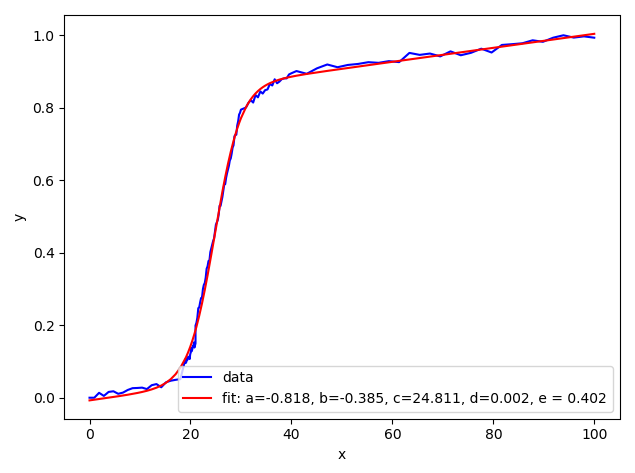H. R. Sheikh, M. F. Sabir, and A. C. Bovik, “A statistical evaluation of recent full reference image quality assessment algorithms,”IEEE Trans. Image Process., vol. 15, no. 11, pp. 3440–3451, Nov. 2006.

展开全文python
• ## Origin曲线拟合教程

千次阅读 2022-07-18 16:39:03机器学习 人工智能 线性回归
• 如何使用Matlab编程进行参数拟合 1前言2基本概念和原理3主要内容4实例5涉及的文件1前言之前帮疯学网做过一个利用Matlab编程进行参数拟合 的教程，由于疯学网好像倒闭了，希望之前做的工作不要白费，这里...
• 时间：2017-03-11 06:45:46声明：欢迎转载，不过记得注明出处哦~前言数据拟合中，最常用的两个就是曲线拟合(curve fitting)与分布拟合(distribution fitting)，不求面面俱到，只希望串出一个思路，篇目如下：3)曲线...
• 1、股票预测问题 已知一只股票在2016年8月每个交易日的收盘价如下表所示，试预测其后面的大体走势。 x = [2 3 4 5 8 9 10 11 12 15 16 17 18 19 22 23 24 25 26 29 30];...p = polyfit(x,y,3) %三次多项式拟合matlab
• 本文实例讲述了Python基于最小二乘法实现曲线拟合。分享给大家供大家参考，具体如下：这里不手动实现最小二乘，调用scipy库中实现好的相关优化函数。考虑如下的含有4个参数的函数式：构造数据import numpy as np ...
• 采用vb.net 2010 对给予的xy点进行曲线拟合，最多可以19次方，与 matlab的 polyfit和polyval的结果基本一致。
• 第 卷 第 期以拍 年 月 北京工 业职业技术学院学报」 价 侧 阳 凭 卫 南 以为 基于 实验数据的曲线拟合与分析 陈金英 史利娟 北京工业职业技术学院 , 北京 《 风 摘 要 通过对 力由 编程 实现实验数据的 曲线拟合 , ...
• 曲线拟合数值方法求解，主要是利用最小二乘法及其线性化法、样条函数插值、三角多项式以及贝塞尔函数法进行拟合，并分析它们的原理以及应用场景。然后，分析各算法流程和核心计算代码模块组织结构，然后对各算法的...机器学习
• Matlab做曲线拟合所给出的表达式参数只有四位有效数字，在有些问题中，在需要用拟合出的表达式进行绘图时，函数对系数对依赖程度过高导致用polt画出对图是折线（类似于将各点坐标连接起来）。若要对绘制曲线进行光滑...
• ## 曲线拟合(1)——Logistic曲线

万次阅读 多人点赞 2020-08-14 08:43:38
文章目录一、Logistic模型1.1 Logistic方程概述1.2 Logistic方程的性质二、Logistic曲线拟合方法2.1 Logistic曲线初值的选取2.2 Logistic曲线参数拟合方法2.2.1 三点法2.2.2 四点法2.2.3 拐点法2.2.4 误差估计——...
• 对allan方差分析后，个不同斜率的项误差进行了参数拟合
• 我们需要知道手臂状态（位置状态、速度状态）和时间的关系，从而拟合出一条连续（重点）的曲线供机械臂达到目的。这就属于“轨迹规划”内容了。 目录 轨迹规划 Joint space、Actuator space、Cartesian space的...ai 三次多项式 拟合曲线
• 车道线拟合方程： #其中lx，ly都为列表 fl = np.polyfit(lx, ly, 3) # 用3次多项式拟合 pl = np.poly1d(fl) # 求3次多项式表达式 print("车道线方程：", pl) lyy = pl(lx) # 拟合y值python
• 用高阶多项式函数拟合曲线； 用解析解求解两种loss的最优解（无正则项和有正则项）； 优化方法求解最优解（梯度下降，共轭梯度）； 用你得到的实验数据，解释过拟合。 用不同数据量，不同超参数，不同的...
• 与数据插值类似，曲线拟合也是一种函数逼近的方法。 构造函数g(x)去逼近未知函数f(x)，使得误差 δi= g(xi)-f(xi)(i=1,2,3,…,n) 在某种意义下达到最小。 最小二乘法（又称最小平方法）是一种数学优化技术。它通过...
• 系列文章目录 提示：这里可以添加系列文章的所有文章...本文先对**【路径、速度、加速度规划】三次、次、七次多项式曲线拟合算法**做个简单的介绍，具体内容后续再更，其他模块可以参考去我其他文章 提示：以下是本
• 【摘要】试验所得的数据一般为离散的，且不能直接用一般的方程去描述它们，这样给数据的分析和预测带来了极大的麻烦，本文针对股票数据的变化，使用matlab的多项式拟合，求导，以及预测功能，对股票的实时变化及其...
• 本人小白，想请教如何在限定参数范围的情况下进行线性拟合。在MATLAB中，通常解一个多元超定方程组，如A=[1,2,3;2,3,4;3,4,5;4,5,6;5,6,7];b=[40,50,60,70,80]';若要求Z=[z1;z2;z3];只需Z=A\b就可以了。但如果额外需...
• ## 曲线拟合问题讲解

千次阅读 2020-12-31 12:39:47
1曲线拟合问题摘要本文首先对给定数据根据不同要求进行多次直线拟合，分别求得使所拟直线预期值的偏差平方和、绝对偏差总和和最大偏差最小的三类拟合直线，然后再求得二次曲线条件下满足三类要求的二次拟合曲线，...
• 本博客采用python的numpy库，用四种方式实现用多项式拟合曲线机器学习 python
• 今天更新较晚主要还是学业繁忙，学习素材也不是很好找，可能很多同学们都在做数学建模以及应用统计时都会涉及到函数参数拟合的问题，一般最常用的方法是最小二乘法，但是当函数参数很多时，往往去普通最小二乘法迭代...matlab 机器学习
• curve_fit(func, x, y) 返回值: popt: 拟合之后的参数 pcov: 参数的协方差矩阵 参数 func: 用来拟合的函数, 自己定义. 函数是几次, 是什么函数(多项式拟合还是三角函数拟合都行) 待定系数是啥都可以定义, 就是自己写...python matlab 数据挖掘
• 在开发医学分析的软件中经常用到四参数Logistic拟合算法，例如化学发光、定量分析等。找了很久，咨询了很多人都敝帚自珍。我终于找到了ImageJ这个好东东，不过是Java的代码，有一天，我终于把它改成Delphi的了。再一...
• 航空等 工 业中都 需要很 好地 解决 样条 曲线拟合的问 题 . 即对 于给定 的一组 离散的有序 点列 要求连 出适 当的曲线使得保 持光顺 , 而且 要便 于数控 绘图 的数据处 理 , 本 文目的是提供 一 种曲线 拟合 和......